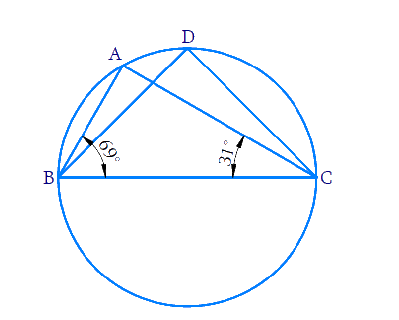In the verge of coronavirus pandemic, we are providing FREE access to our entire Online Curriculum to ensure Learning Doesn't STOP!

# Ex.10.5 Q4 Circles Solution - NCERT Maths Class 9

Go back to  'Ex.10.5'

## Question

In the given figure, \begin {align} \angle {ABC}=69^{\circ} \end {align} and \begin {align} \angle {ACB}=31^{\circ} \end {align}find \begin {align} \angle {BDC} . \end {align}Video Solution
Circles
Ex 10.5 | Question 4

## Text Solution

What is known?

Two angles in a triangle.

What is unknown?

Value of \begin {align} \angle {BDC}\end {align}

Reasoning:

• Sum of angles in a triangle is \begin {align} 180^{\circ}.\end {align}
• Angles in the same segment are equal.

Steps:

Consider the \begin {align} \Delta {ABC,}\end {align} the sum of all angles will be \begin {align} 180^\circ.\end {align}

\begin{align} \angle {ABC}+ \!\!\angle {BAC}+ \!\angle {ACB} &=180^{\circ} \\ 69^{\circ}+\angle {BAC}+31^{\circ} &=180^{\circ} \end{align}

\begin{align} \angle {BAC} &=180^{\circ}-\left(69^{\circ}+31^{\circ}\right) \\ &=180^{\circ}-100^{\circ} \\ &=80^{\circ} \end{align}

We know that, angles in the same segment of a circle are equal.

\begin {align} ∴ \angle {BDC}=\angle {BAC}=80^{\circ} \end {align}

Video Solution
Circles
Ex 10.5 | Question 4

Learn from the best math teachers and top your exams

• Live one on one classroom and doubt clearing
• Practice worksheets in and after class for conceptual clarity
• Personalized curriculum to keep up with school